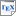[Par02] David Parker. Implementation of Symbolic Model Checking for Probabilistic Systems. Ph.D. thesis, University of Birmingham. 2002. [pdf] [bib] Downloads:pdf (1.51 MB)bib Links: [Google] [Google Scholar] [CiteSeer] Abstract. In this thesis, we present efficient implementation techniques for probabilistic model checking, a method which can be used to analyse probabilistic systems such as randomised distributed algorithms, fault-tolerant processes and communication networks. A probabilistic model checker inputs a probabilistic model and a specification, such as "the message will be delivered with probability 1", "the probability of shutdown occurring is at most 0.02" or "the probability of a leader being elected within 5 rounds is at least 0.98", and can automatically verify if the specification is true in the model. Motivated by the success of symbolic approaches to non-probabilistic model checking, which are based on a data structure called binary decision diagrams (BDDs), we present an extension to the probabilistic case, using multi-terminal binary decision diagrams (MTBDDs). We demonstrate that MTBDDs can be used to perform probabilistic analysis of large, structured models with more than 7.5 billion states, way out of the reach of conventional, explicit techniques, based on sparse matrices. We also propose a novel, hybrid approach, combining features of both symbolic and explicit implementations and show, using results from a wide range of case studies, that this technique can almost match the speed of sparse matrix based implementations, but uses significantly less memory. This increases, by approximately an order of magnitude, the size of model which can be handled on a typical workstation.